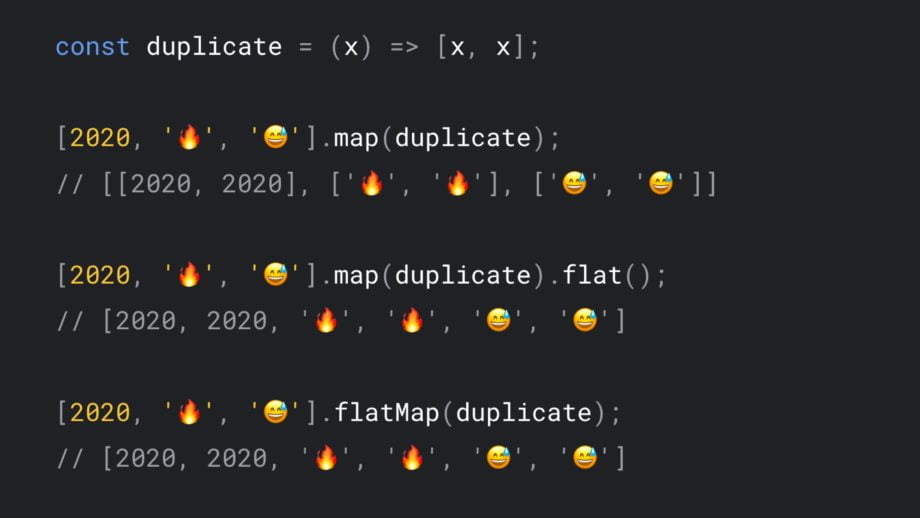# Flatten Arrays in Vanilla JavaScript with flat() and flatMap()

Share

ES2019 introduced two methods on the array prototype that would make life so much simpler for developers. These are flat() and flatmap() which help in flattening arrays.

## Array.prototype.flat()

As the name suggests, the flat() method returns a new array with elements of the subarray flattened into it, that is the sub-elements are concatenated into a single array.

``````[[1, 2], [3, 4], [5, 6]].flat();

// [1, 2, 3, 4, 5, 6]``````

By default, it flattens only one level deep, but it does take a depth argument which can be used to specify how many levels of depth we want to flatten.

``````const twoLevelsDeep = [[1, [2, 2], 1]];

// depth = 1
twoLevelsDeep.flat();
// [1, [2, 2], 1]

// depth = 2
twoLevelsDeep.flat(2);
// [1, 2, 2, 1]
``````

Note: Infinity is a valid value for the depth if we want it to go all the way down.

## Array.prototype.flatMap()

flatMap is a smarter version of map, which does mapping of an array and flattening in one go. It does map() first, and then flat() even though that is not the order in the naming of the function.

``````const newArray = arrayObject.flatMap(callback(currentValue, index, array) {
...
}, thisArg);``````

As can be seen, the flatMap method takes in a callback function and a reference to this as arguments. The thisArg is optional and is the value used for the “this” variable inside the callback.

The callback function contains a reference to the current value and the index and array values are optional. The return value of the function is the flattened value of the array returned by the callback function.

This is a very common operation with nested data where you want to select children from an array of objects. But before that, let us look at a contrived example:

``````let array = [1, 2, 3, 4];

array.map(x => [x * 2]);
// [, , , ]

array.flatMap(x => [x * 2]);
// [2, 4, 6, 8]

// only one level is flattened
array.flatMap(x => [[x * 2]]);
// [, , , ]``````

There is no way to specify the depth in a flatMap and it is set to 1 by default.

A more practical use case would be, if we wanted to check all the roles all users have:

``````[
{ name: "Saransh Kataria" , roles: ['system-admin', 'developer'] },
{ name: "Wisdom Geek" , roles: ['basic'] },
].flatMap(x => x.roles);

// Output => ["system-admin", "developer", "basic"]``````

It is also possible to add or remove elements when using flatMap() which is not a possibility using map().

If we wanted to remove all negative items from an array:

``````const numbers = [-1, 2, 3, -4, 5];

numbers.flatMap(number => {
return number < 0 ? [] : [number];
});

// [ 2, 3, 5]``````

It can act as filter because of the empty array.

``````const emptyNestedArray = [[], 1];

emptyNestedArray.flat();
// [ 1 ]``````

Similarly, if we return an array with multiple elements from the callback, those would be added as individual items to the flattened array.

For example, if we wanted to add a number’s double and triple right after every element in an array:

``````const numbers = [1, 2];
const appendedDoublesTriples = numbers.flatMap(number => {
return [number, 2 * number, 3 * number];
});
console.log(appendedDoublesTriples);
// logs [1, 2, 3, 2, 4, 6]``````

And that is all there is to know about flat and flatMap(). Hope these were helpful and again, these were a part of ES2019, so we need to add polyfills if we are supporting browsers before that.